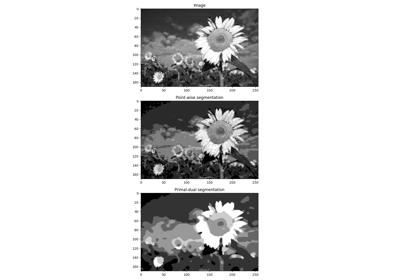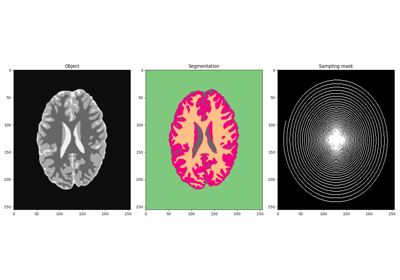# pyproximal.optimization.segmentation.Segment#

pyproximal.optimization.segmentation.Segment(y, cl, sigma, alpha, clsigmas=None, z=None, niter=10, x0=None, callback=None, show=False, kwargs_simplex=None)[source]#

Primal-dual algorithm for image segmentation

Perform image segmentation over $$N_{cl}$$ classes using the general version of the first-order primal-dual algorithm .

Parameters
ynp.ndarray

Image to segment (must have 2 or more dimensions)

clnumpy.ndarray

Classes

sigmafloat

Positive scalar weight of the misfit term

alphafloat

Positive scalar weight of the regularization term

clsigmasnumpy.ndarray, optional

Classes standard deviations

znumpy.ndarray, optional

niterint, optional

Number of iterations of iterative scheme

x0numpy.ndarray, optional

Initial vector

callbackcallable, optional

Function with signature (callback(x)) to call after each iteration where x is the current model vector

showbool, optional

Display iterations log

kwargs_simplexdict, optional

Arbitrary keyword arguments for pyproximal.Simplex operator

Returns
xnumpy.ndarray

Classes probabilities. This is a vector of size $$N_{dim} \times N_{cl}$$ whose columns contain the probability for each pixel to be in the class $$c_i$$

clnumpy.ndarray

Estimated classes. This is a vector of the same size of the input data y with the selected classes at each pixel.

Notes

This solver performs image segmentation over $$N_{cl}$$ classes solving the following nonlinear minimization problem using the general version of the first-order primal-dual algorithm of :

$\min_{\mathbf{x} \in X} \frac{\sigma}{2} \mathbf{x}^T \mathbf{f} + \mathbf{x}^T \mathbf{z} + \frac{\alpha}{2}||\nabla \mathbf{x}||_{2,1}$

where $$X=\{ \mathbf{x}: \sum_{i=1}^{N_{cl}} x_i = 1,\; x_i \geq 0 \}$$ is a simplex and $$\mathbf{f}=[\mathbf{f}_1, ..., \mathbf{f}_{N_{cl}}]^T$$ with $$\mathbf{f}_i = |\mathbf{y}-c_i|^2/\sigma_i$$. Here $$\mathbf{c}=[c_1, ..., c_{N_{cl}}]^T$$ and $$\mathbf{\sigma}=[\sigma_1, ..., \sigma_{N_{cl}}]^T$$ are vectors representing the optimal mean and standard deviations for each class.

1(1,2)

Chambolle, and A., Pock, “A first-order primal-dual algorithm for convex problems with applications to imaging”, Journal of Mathematical Imaging and Vision, 40, 8pp. 120–145. 2011.

## Examples using pyproximal.optimization.segmentation.Segment#Image segmentation

Image segmentationMRI Imaging and Segmentation of Brain

MRI Imaging and Segmentation of Brain